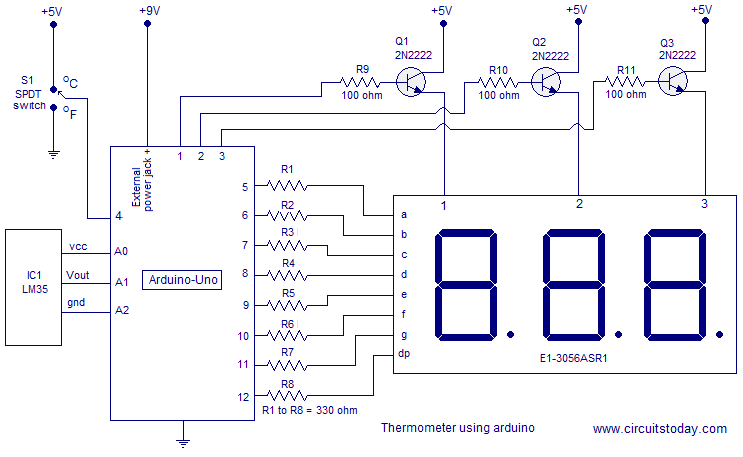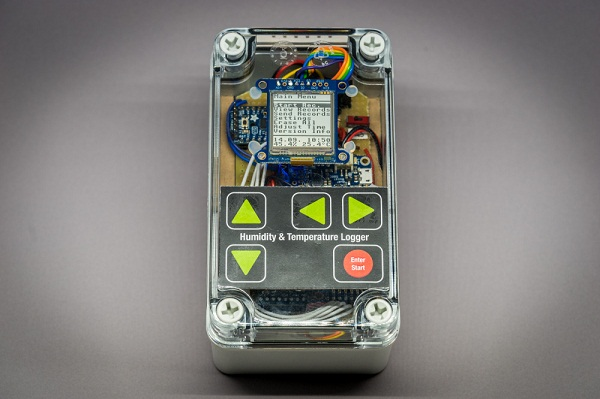# Digital thermometer using arduino and LM35

This article is about a simple three digit digital thermometer using arduino. Range of this thermometer is from 0°C to 99.9°C. There is also a provision for displaying the temperature in °F scale. Three terminal analog temperature sensor LM35 is used as the sensor here. LM35 can measure temperatures between -55°C to +155°C. The supply voltage range is from 4V to 30V DC and the current drain is 60uA. The LM35 is available in TO-92 package and it is very easy to use. The output voltage of the arduino increases 10mV per °C rise in temperature. That means if 25 °C is the temperature, then output  voltage of the sensor will be 250mV. Circuit diagram of the digital thermometer using arduino and LM35 is shown in the figure below.

#### Circuit diagram.Temperature sensor LM35 is connected to the arduino through the analog input pins. A0 pin of the arduino serves as the supply voltage source for LM35 and A2 pin of the arduino serves as the ground. Arduino reads the voltage output of the LM35 through the analog input pin A1. Digital  pin 4 is used for interfacing the °C/°F selector switch to the arduino. Digit driver transistors Q1, Q2 and Q3 are interfaced to the digital pins 1, 2 and 3 of the arduino respectively. Multiplexed segment lines  a to dp are interfaced to the digital pins 5 to 12 of the arduino respectively. Resistors R9, R10 and R11 limits the base current of the corresponding transistors. Resistors R1 to R8 limits the current through the corresponding segments.

READ  Pimp My Zoomobil using Arduino

#### This Post / Project can also be found using search terms:

• digital thermometer lm35 arduino
• Digital Thermometer using only an arduino board and LM35 no LCD
• PRINCIPLE OF DIGITAL THEMAMETER WITH ARDUINO BY LM35